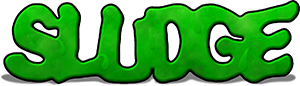## Passing Functions as Variables

A variable can be used to hold a number, a line of text, an object type... and a function. This is useful if you have a large number of functions which should all be called in the same way... for example, the functions which are used to set up the scenes of your game.

Rather than having a program defined as follows...

```var lastRoom;      # The room we just came from (string)
var thisRoom;      # The room we're in now (string)

sub goToDiningRoom () {
removeAllCharacters ();
removeScreenRegions ();
lastRoom = thisRoom;
thisRoom = "dining room";
completeTimers ();

addOverlay ('diningRoom.tga', 0, 0);
setFloor ('diningRoom.flo');
# More dining room initialisation here
}

sub goToLounge () {
removeAllCharacters ();
removeScreenRegions ();
lastRoom = thisRoom;
thisRoom = "lounge";
completeTimers ();

addOverlay ('lounge.tga', 0, 0);
setFloor ('lounge.flo');
# More lounge initialisation here
}```

It would be beneficial to have a number of rooms and one function for changing between them.

```var lastRoom;      # The room we just came from (function handle)
var thisRoom;      # The room we're in now (function handle)

sub goToRoom (newRoom) {
removeAllCharacters ();
removeScreenRegions ();
lastRoom = thisRoom;
thisRoom = newRoom;
completeTimers ();
newRoom ();
}

sub diningRoom () {
addOverlay ('diningRoom.tga', 0, 0);
setFloor ('diningRoom.flo');
# More dining room initialisation here
}

sub lounge () {
addOverlay ('lounge.tga', 0, 0);
setFloor ('lounge.flo');
# More lounge initialisation here
}```

You can see that, at the end of the goToRoom sub, newRoom can be called just like a function even though it's actually a variable. This is because if your program was to include a line such as

`goToRoom (lounge);`

...the value of newRoom would be a pointer to the lounge function. Therefore, the lounge function would be called. You can even pass pointers to functions which take variables, although there are probably very few situations where you would want to...

```sub doRepeatingMaths (number, mathFunc, mathSign) {
var total = number;
var newTotal;

for (var a = 0; a < 5; a ++) {
newTotal = mathFunc (total, number);
say (ego, total + mathSign + number + " = " + newTotal);
total = newTotal;
}
}

sub minus (a, b)   { return a - b; }

sub plus (a, b)   { return a + b; }

sub times (a, b)   { return a * b; }```

Using the above code, a call to doRepeatingMaths (2, plus, "+") would make the ego character say the following:

2+2 = 4

4+2 = 6

6+2 = 8

8+2 = 10

10+2 = 12

A call to doRepeatingMaths (3, times, "*") would make the ego character say:

3*3 = 9

9*3 = 27

27*3 = 81

81*3 = 243

243*3 = 729